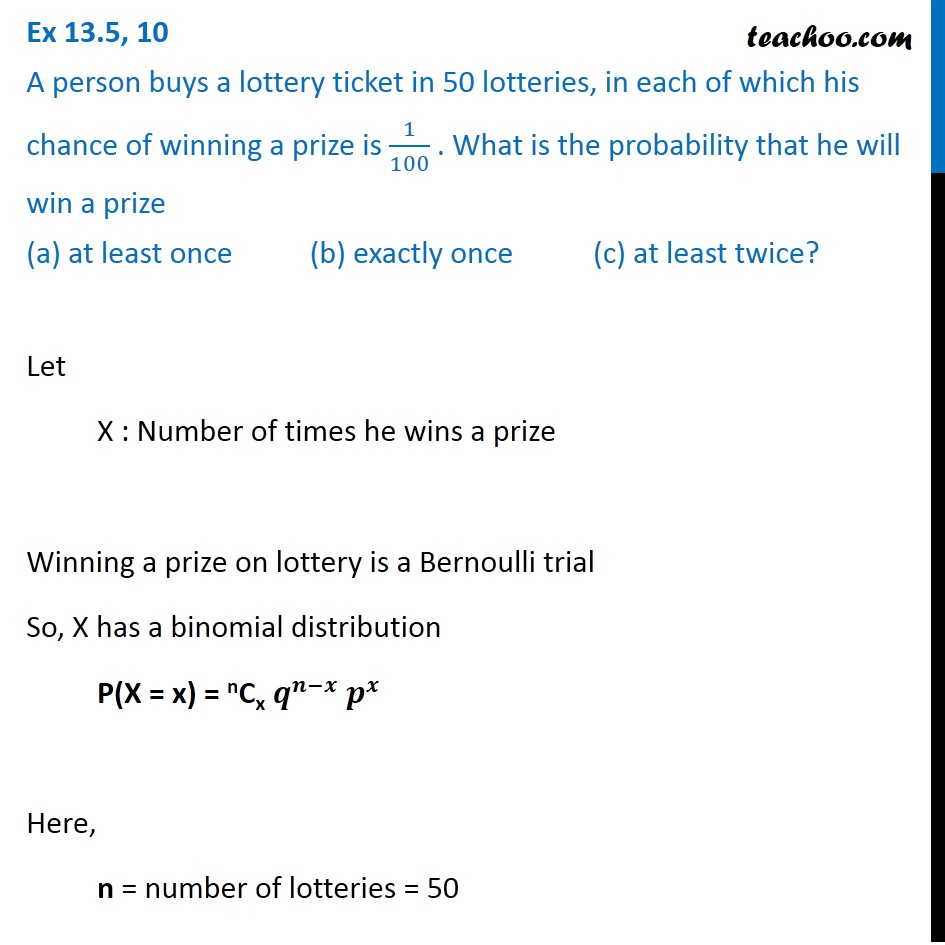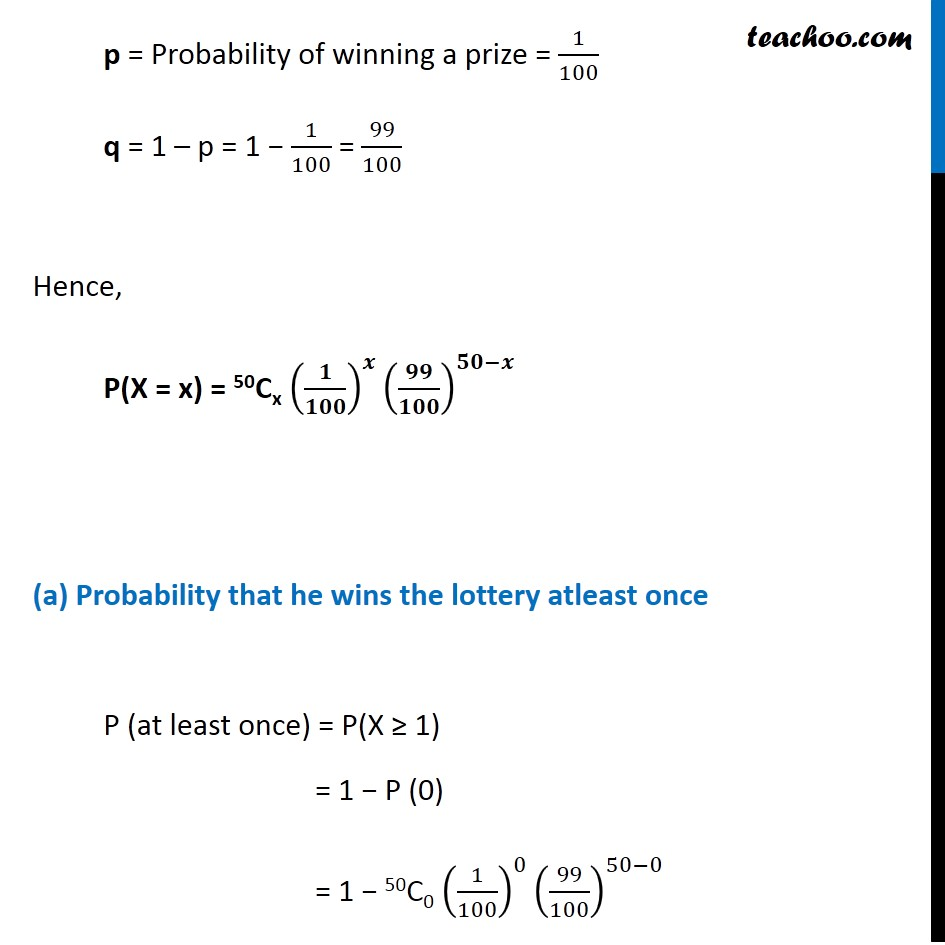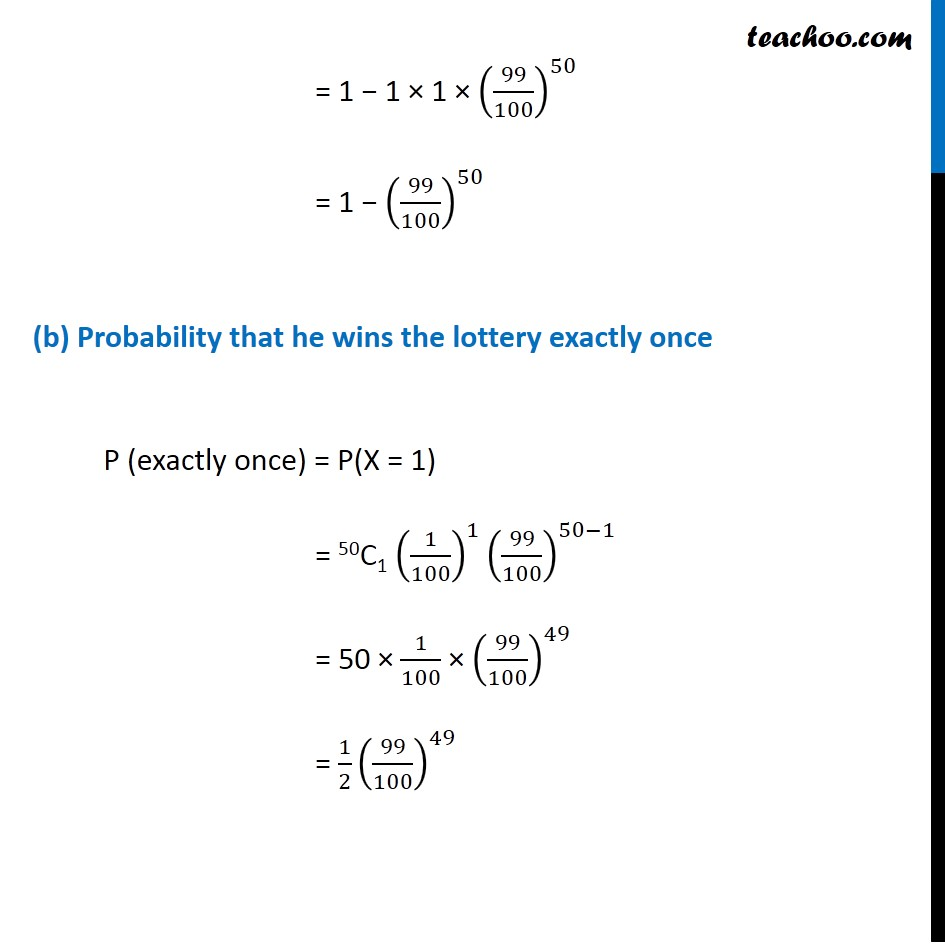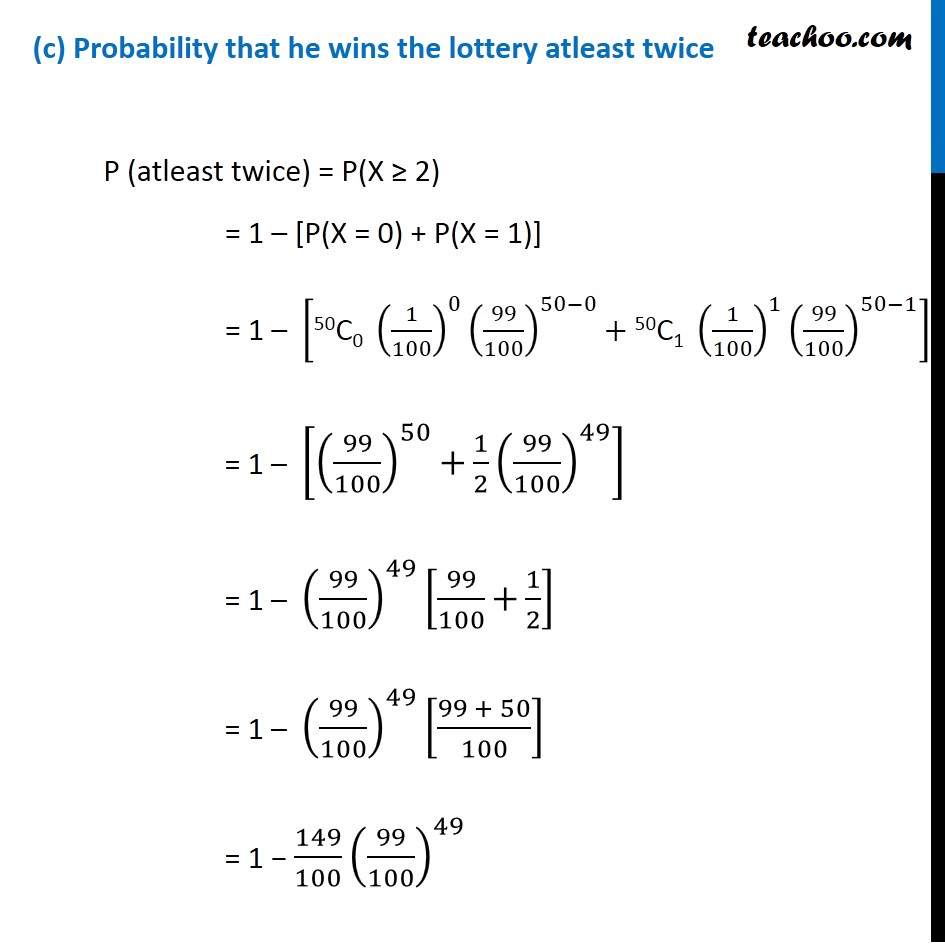Bernoulli Trial

Chapter 13 Class 12 Probability
Serial order wiseLearn in your speed, with individual attention - Teachoo Maths 1-on-1 Class

### Transcript

Question 10 A person buys a lottery ticket in 50 lotteries, in each of which his chance of winning a prize is 1/100 . What is the probability that he will win a prize (a) at least once (b) exactly once (c) at least twice?Let X : Number of times he wins a prize Winning a prize on lottery is a Bernoulli trial So, X has a binomial distribution P(X = x) = nCx 𝒒^(𝒏−𝒙) 𝒑^𝒙 Here, n = number of lotteries = 50 Let X : Number of times he wins a prize Winning a prize on lottery is a Bernoulli trial So, X has a binomial distribution P(X = x) = nCx 𝒒^(𝒏−𝒙) 𝒑^𝒙 Here, n = number of lotteries = 50 p = Probability of winning a prize = 1/100 q = 1 – p = 1 − 1/100 = 99/100 Hence, P(X = x) = 50Cx (𝟏/𝟏𝟎𝟎)^𝒙 (𝟗𝟗/𝟏𝟎𝟎)^(𝟓𝟎−𝒙) (a) Probability that he wins the lottery atleast once P (at least once) = P(X ≥ 1) = 1 − P (0) = 1 − 50C0 (1/100)^0 (99/100)^(50−0) = 1 − 1 × 1 × (99/100)^50 = 1 − (99/100)^50 (b) Probability that he wins the lottery exactly once P (exactly once) = P(X = 1) = 50C1 (1/100)^1 (99/100)^(50−1) = 50 × 1/100 × (99/100)^49 = 1/2 (99/100)^49 (c) Probability that he wins the lottery atleast twice P (atleast twice) = P(X ≥ 2) = 1 – [P(X = 0) + P(X = 1)] = 1 – ["50C0 " (1/100)^0 (99/100)^(50−0) "+ 50C1 " (1/100)^1 (99/100)^(50−1) ] = 1 – [(99/100)^50 "+" 1/2 (99/100)^49 ] = 1 – (99/100)^49 [99/100 "+" 1/2] = 1 – (99/100)^49 [(99 + 50)/100] = 1 − 149/100 (99/100)^49